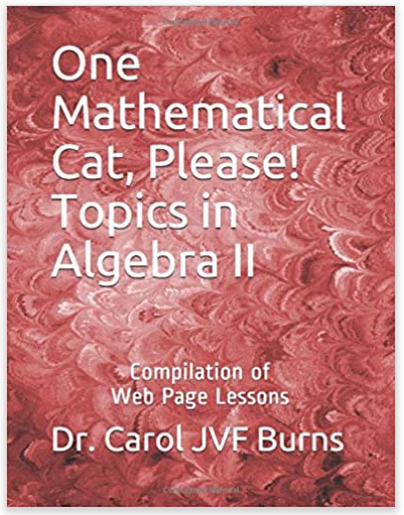# ONE MATHEMATICAL CAT, PLEASE! Topics in Algebra IIGet a print version of these lessons.

This book is printed in black-and-white. The print size is small, since each lesson matches the web page exactly, and is then shrunk to fit an 8.5" x 11" page. Check the Amazon preview. Young eyes only!
Section 1:
Recursion, Sequences, and Applications
Section 2:
Functions and their Graphs
7. graphical interpretation of sentences like $\,f(x) = 0\,$ and $\,f(x)\gt 0\,$
8. graphical interpretation of sentences like $\,f(x) = g(x)\,$ and $\,f(x) \gt g(x)\,$
Section 3:
Probability
Section 4:
Statistics
Section 5:
Systems of Equations
Section 6:
Matrices
Section 7:
Graphical Transformations
27. vertical and horizontal translations (moving up, down, left, and right)
28. vertical and horizontal scaling (stretching and shrinking)
Section 8: